# Overview

• Motivation
• How to create Web report
• LTSPICE RC Circuit
• LTSPICE_Operating_Point_Analysis
• LTSPICE_AC_Analysis
• LTSPICE_Transient_Analysis
• ADC_DAC_Ramp_Simulation
• DAC_Data_Analysis
• ADC_Sine_Data_Analysis
• ADC_Sine_FFT
• R2R_DAC
• R2R_DAC_Sine FFT
• R2R_DAC_Ramp
• Extracting Data from LTSPICE

• Work distribiution:
• Including ADC_DAC_Ramp_Simulation: ***742R
• From there: ***136H

# Motivation

• This laboratory series is about creating a webreport, unstanding LTSpice simulation. Furthermore we ananalyzed ideal and non ideal ADC/DAC circuit with ramp and sine input voltage. Therefore INL/DNL and FFT has been calculated with an online tool.

# How to create web report

• Use template which is available
• here
• Change: Title, Name, slide footer
• Copy div class "slide" for slide content
• Content in div class "handout" is not on the slides displayed
• Insert Images like this: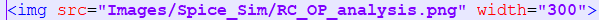• Insert LTSpice Schematic like this: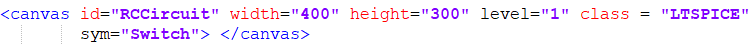• -> Spice operation commands are not displayed with this method

# LTSPICE_RC_Circuit

## Creating a Circuit

 Insert Components Set component values Add Net Labels Add simulation comand Set background color to white to have good visible results: "tools -> color preferences"

# LTSPICE_Operating_Point_Analysis

 use following command: .op No DC current through capacitance Not very usefull in an AC filter circuit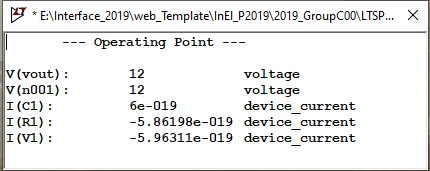# LTSPICE_AC_Analysis

 use following command: .ac dec 10 10 1k Simulation 10Hz to 1kHz Corner frequ. ~32Hz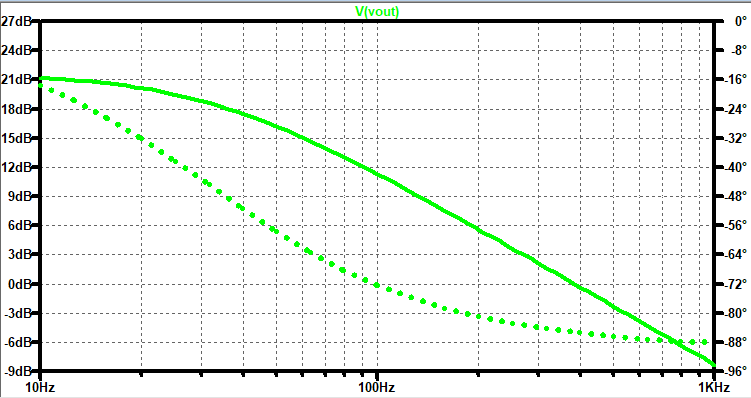# LTSPICE_Transient_Analysis

 use following command: .tran 20m AC Source with 100Hz Simulation 20ms -> 2 periods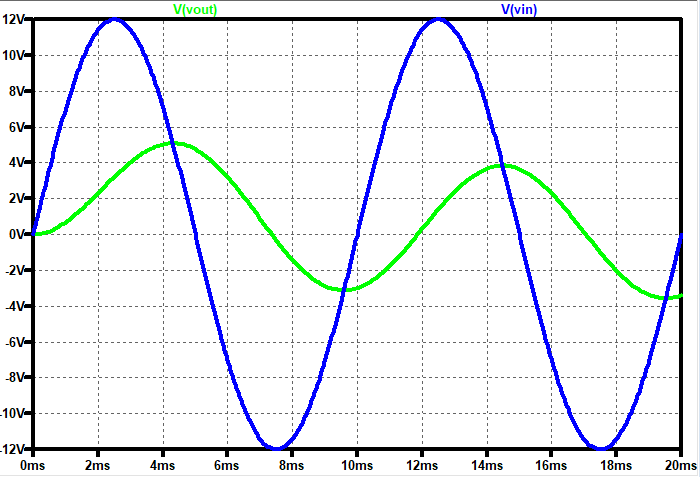# ADC_DAC_Ramp_Simulation

 Download all Symbol and Schematic files frome here Add an DC Source for a Ramp Simulation: V1 in1 0 PULSE(0 1 0 655.36u 655.36u 0 131.072u ) Rename V2 "in1" in "nx1" because two parallel voltage sources are not allowed Run the simulation Hint: To reduce the .RAW file size delete "V(V1)" in the .save command and change the source "VCLK" to "VCLK CLK 0 PULSE(0 1 0 1n 1n 79n 160n)" to have less steps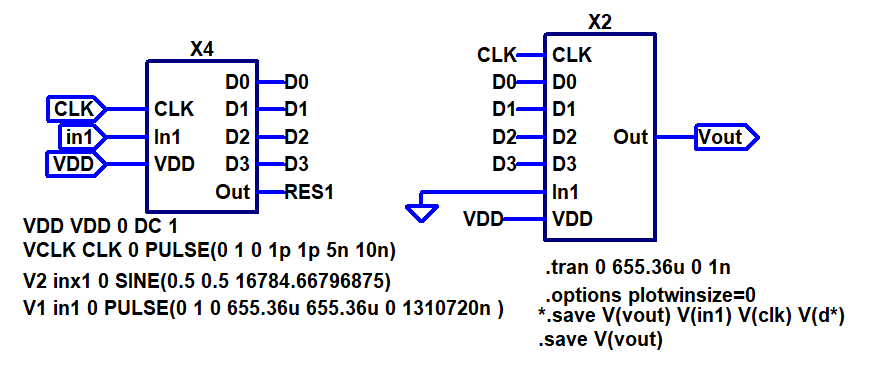# ADC_DAC_Ramp_Simulation

 Result: The ramp voltage at the input is converted to an digital signal and the digital signal is converted to the analog stair case curve It is a 4-bit ADC so max voltage is 1V/16*(16-1)=0,94V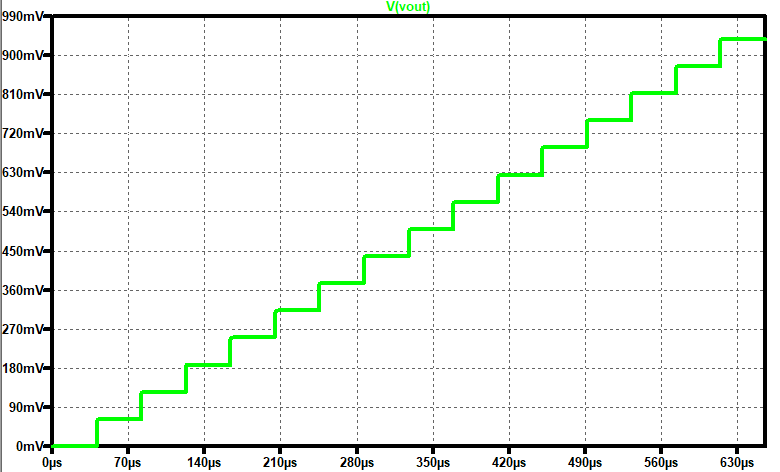# DAC_Data_Analysis

 Use this tool for filtering the data. Adjust "End_time" to Spice Simulation time For DAC analysis we need one datapoint for each stair Adjust "Time Step" to the value "End_time"/"number of DAC stairs" You will get one Data-Point for every stair -> Thats what you need for DAC INL/DNL analysis After map to 15 integer values you get a curve like figure 1 The DNL / INL in figure 2 shows the ideal DAC, there is no diviation to the ideal curve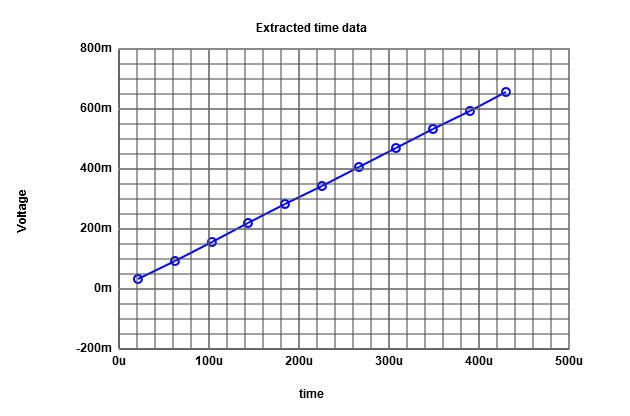figure1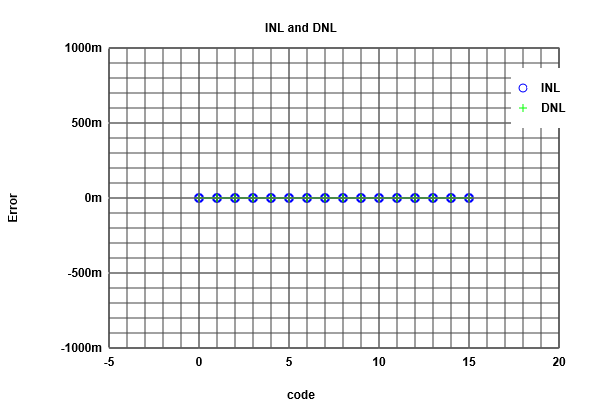figure2

# ADC_Sine_Data_Analysis

 For ADC analysis we are interested how large the stairs are Adjust "Time Step" to 5.12e-6 so we get 8 Datapoints each ADC step After map to 15 integer values you get a curve like figure 1 All steps have the same size -> ideal ADC Now for the ADC we can do a histogram figure 2 -> no error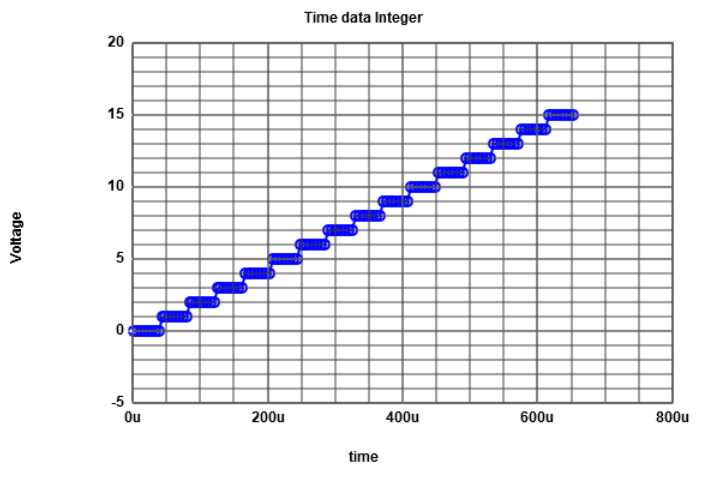figure1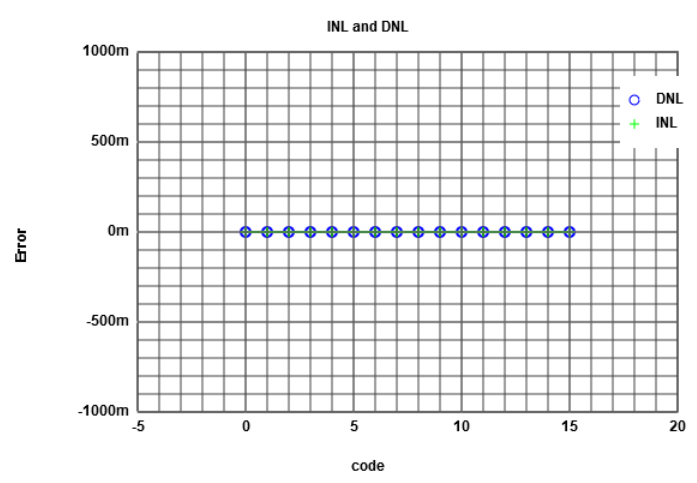figure2

# ADC_Sine_Data_Analysis

 Now we do the simulation again with a sinusoidal input voltage In practice a high quality sine wave is better to generate than an perfect ramp Adjust "Time Step" to 5.12e-6 so we get 8 Datapoints each ADC step After map to 15 integer values you get a curve like figure 1 Now for the ADC we can do a histogram figure 2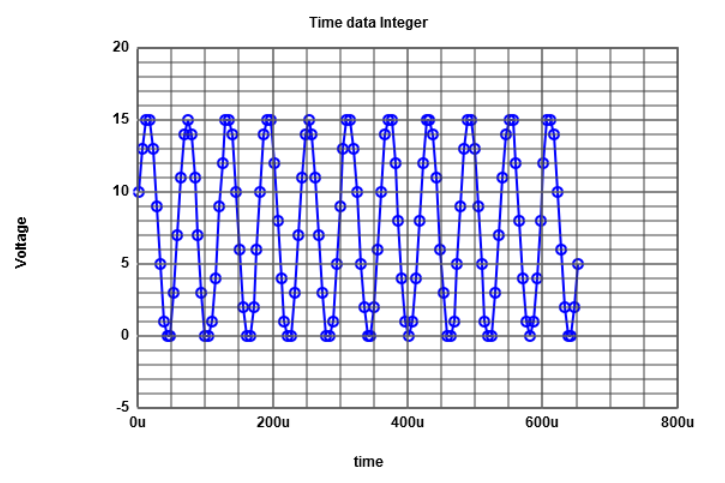figure1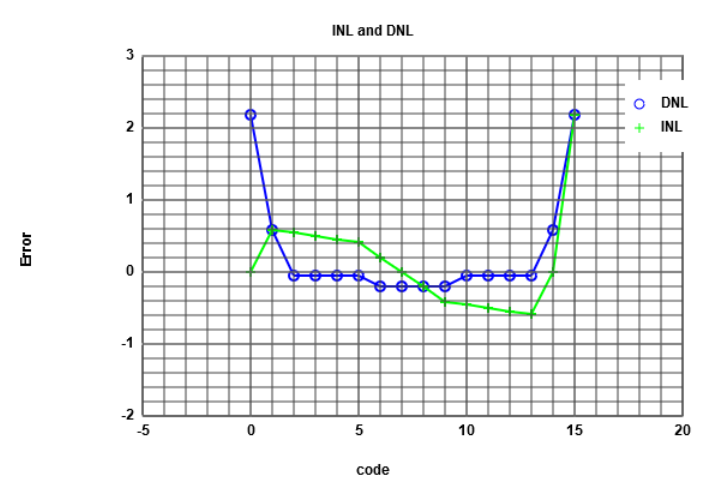figure2

# ADC_Sine_FFT

 Copy the mapped data points into this page to calculate a FFT Adjust the number of points and set number of bits to 4 You see the calculated FFT in figure 1 The you can read in figure 1 the SNR is ~24dB In figure 2 you see the INL/DNL with a real sine as reference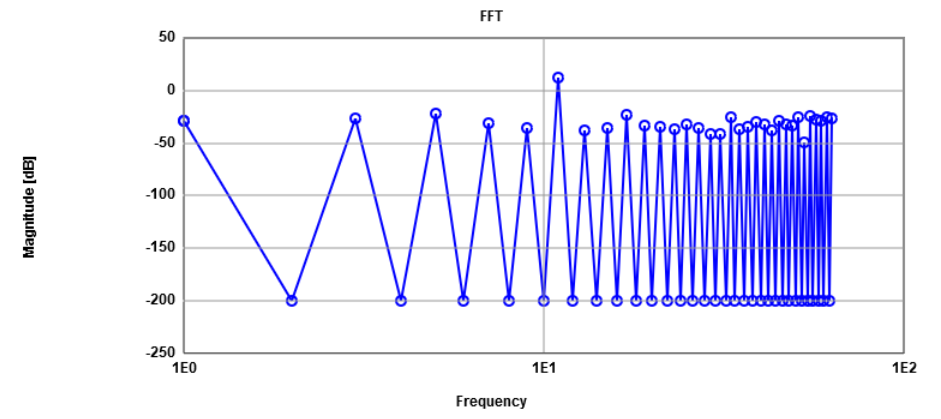figure1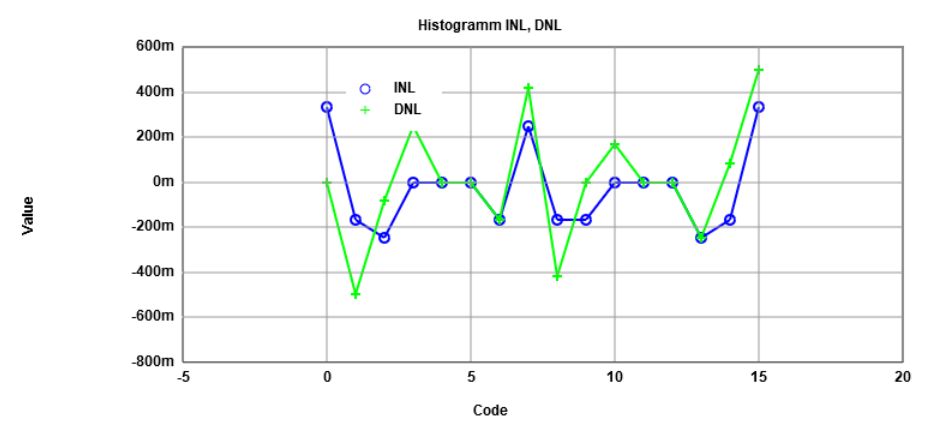figure2

# R2R_DAC

 Download R2R_DAC here Insert the R2R circuit in ADC_DAC_pipe Rename the output of the ideal DAC to Voutx (voltages with same name are not allowed) Change R9 to 1.5 and R6 to 1.3k to simulate a non ideal DAC

# R2R_DAC_Sine

 Figure 1 shows the ideal R2R DAC with sine input The highest changes are where the sine has it's highest slop We see we got a "big" jump arround 0.5V in figure 2 because we changed the values of the two higher "bit" resistors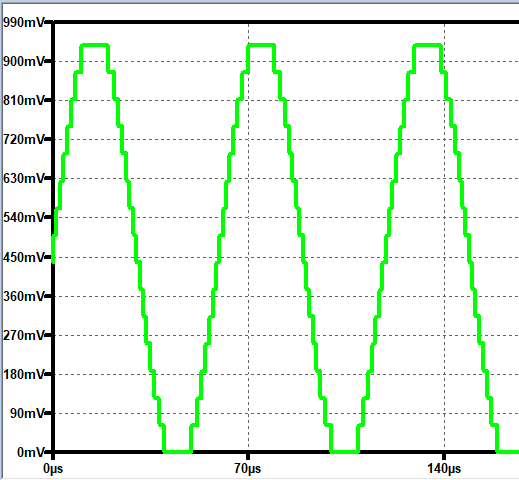figure1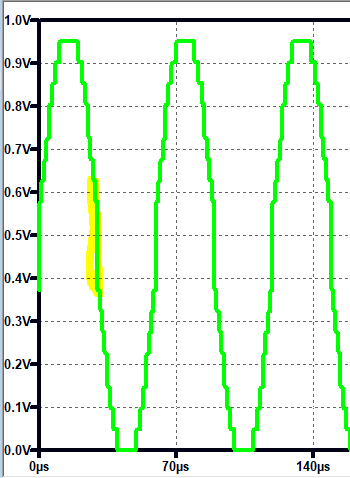figure2

# R2R_DAC_Sine FFT

 In figure 1 we see the FFT of the non ideal DAC The SNR decreased to 18dB In figure 2 we see the histogram We have now errors greater than one -> ADC has less precision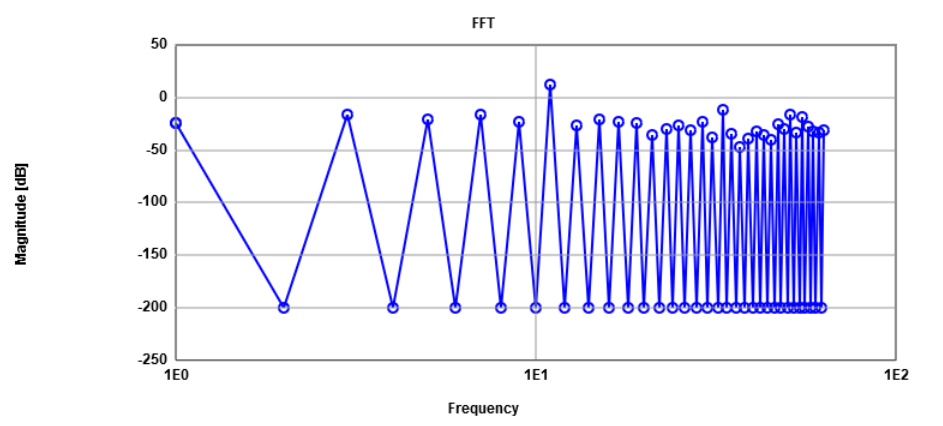figure1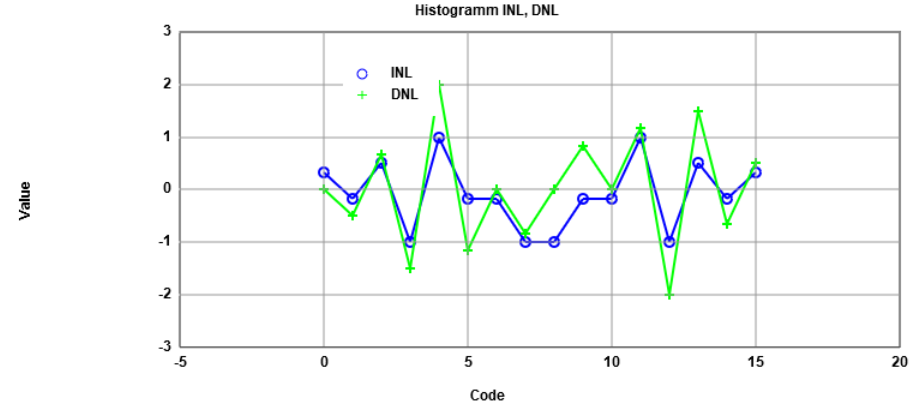figure2

# R2R_DAC_Ramp

 Select the ramp voltage source in LTSPICE Figure 1 shows the ideal R2R DAC (green) and the simulated data (blue) Figure 2 shows two peaks due to the error of the lower bit resistor and one high peak due to the highest order bit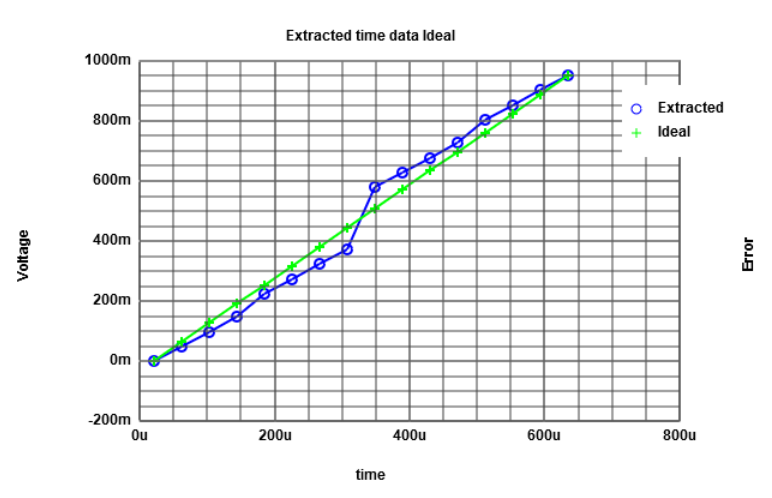figure1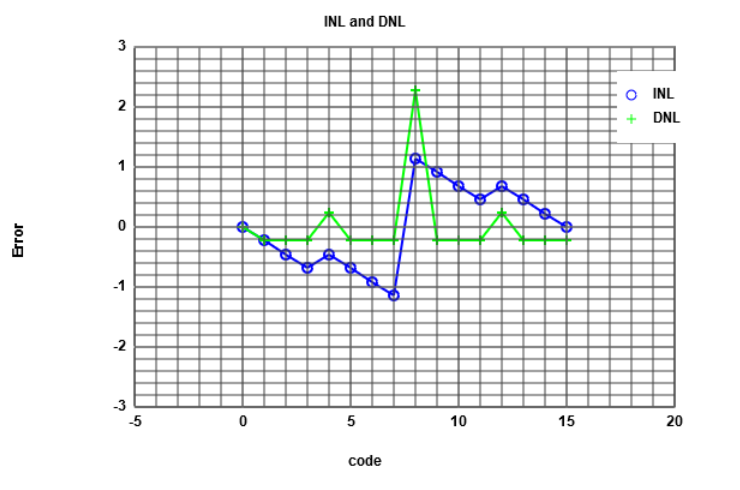figure2

# Extracting Data from LTSPICE

• For extracting Data from LTSPICE you can either use the .js tool we used the slides before
• Or you can use the .meas statement
• Insert in the DAC_pipe following command:
".meas trans OUT00 FIND V(vout) AT = 81.92us"
• The voltage source "vout" will be measured at simulation time 81.92us
• To see the measured data: "View"->"Spice Error Log"
• You will get high number of measuring points with when simulating DAC with more bits